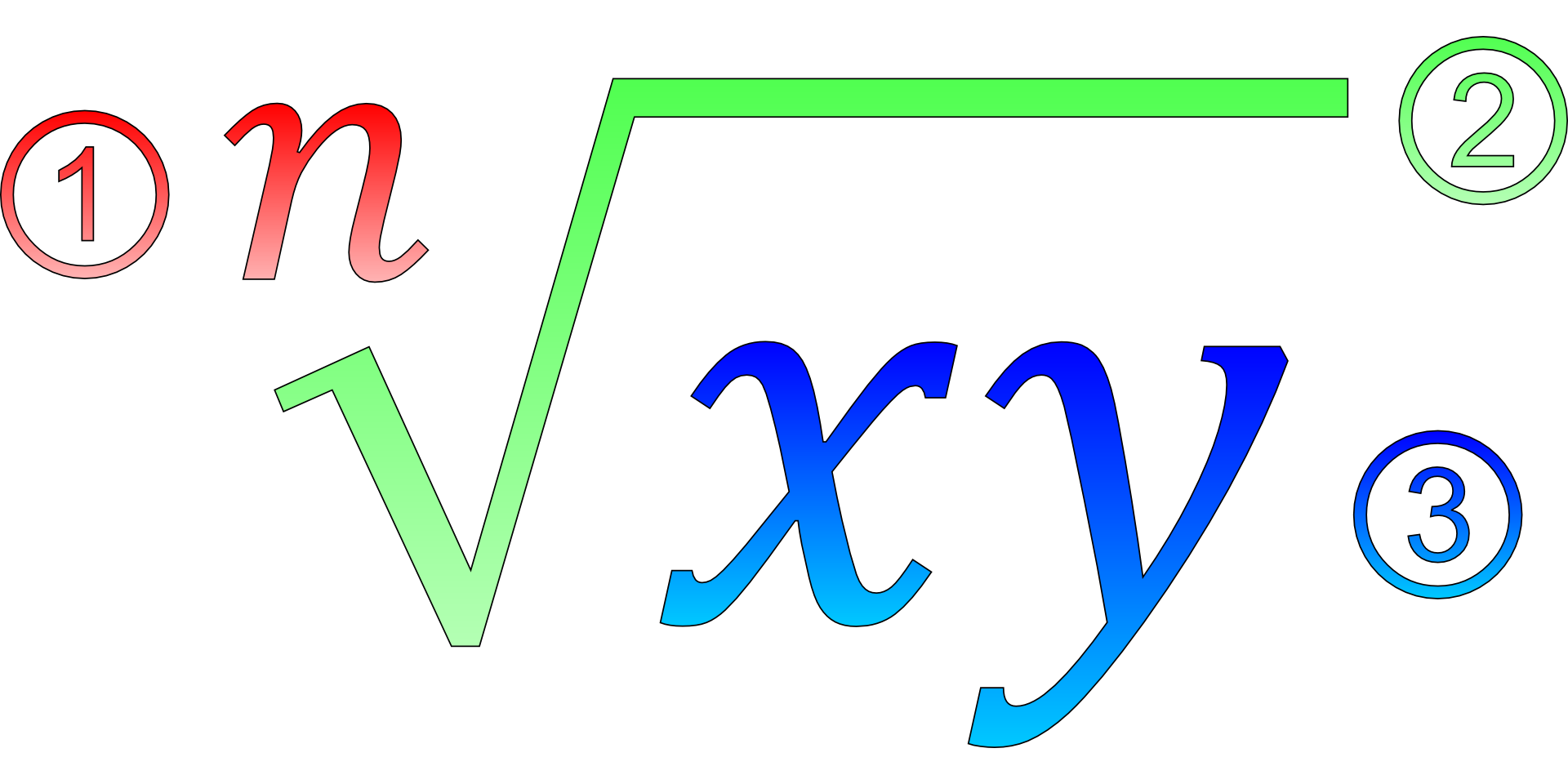# Algebra 2\$60.00
\$100.00

Dex Math provides a complete online Algebra 2 course. Perfect for students who need to catch up on their Algebra 1 skills, we also offer a personalized tutoring and homework assistance for your kids needs.

Algebra 2 is a high school math course exploring how to use letters (called variables) and numbers with mathematical symbols to solve problems.

Algebra 2 typically includes evaluating expressions, writing equations, graphing functions, solving quadratics, and understanding inequalities. Algebra 1 focuses on a variety of different types of functions such as linear, quadratic, and cubic — all of which are represented in traditional “function notation” as well as graphed on the coordinate plane.

Students in Algebra 2 typically build conceptual understanding and gain procedural fluency of concepts that are required for more advanced math subjects such as Geometry and Calculus. Abstracting real-life situations into mathematical models (expressions, equations, functions) is a key part of success in Algebra 1.

What You'll Learn

• Equations and inequalities
• How to graph functions and linear equations
• How to solve system of linear equations
• Matrices